# Type-1-Problems on MI of simple figures

1.Determine the MI of a triangular section having base 5 cm and 6 cm height about its base.

{Ans: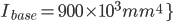2.A triangular section has base 100 mm and 300 mm height determine moment of inertia about 1)MI about axis passing through base 2)MI about axis passing through apex

{Ans: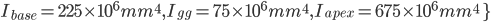3.Find the moment of inertia of a hollow circular section having external diameter 100 mm and internal diameter 80mm about,

1) Axis passing through center 2) About tangent to the outer circle and parallel to xx axis.

{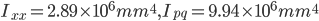}

4.Find the moment of inertia of a hollow rectangular section about its centre of gravity, if the external dimensions are 40 mm deep and 30 mm wide and internal dimension are 25 mm and 15 mm wide.

{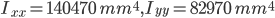}

5. An isosceles triangular section ABC has base width 80 mm and height 60mm. Determine the moment of inertia of the section about the centre of gravity of the section and the base BC.

{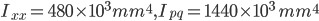}

6.A hollow C.I. pipe with external diameter 100 mm and thickness of metal 10 mm is used as a strut. Calculate the moment of inertia and radius of gyration about its diameter.

{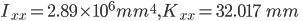}

7.A circular disc has M.I. about its any tangent is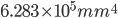. Find the diameter of the disc.

{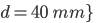8.An equilateral triangle has a side of 150 mm. Find the moment of inertia about any of its sides.

{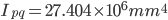}

9.Find MI of an equilateral triangle of side 2m about its base.

{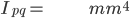}

10.A semicircular lamina has a base diameter 140mm. Calculate the moment of inertia

{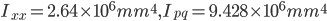}

11.Calculate Polar MI of a square section having 200mm as side.

{ }

12.Calculate polar moment of inertia for a circle having diameter 250 mm.

{ }